### Example 7.3 Using the INEST=Option

This example illustrates the use of the INEST= option for specifying a starting point and linear constraints. You name a data set with the INEST= option. The format of this data set is similar to the format of the QUAD data set described in the previous example.

Consider the Hock and Schittkowski (1981) Problem # 24: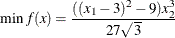subject to: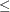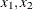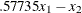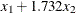with minimum function value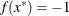at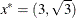. The feasible starting point is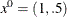.

You can specify this model in PROC NLP as follows:

proc nlp tech=trureg outest=res;
min y;
parms x1 = 1,
x2 = .5;
bounds  0 <= x1-x2;
lincon  .57735 * x1 -         x2 >=  0,
x1 + 1.732 * x2 >=  0,
-x1 - 1.732 * x2 >= -6;
y = (((x1 - 3)**2 - 9.) * x2**3) / (27 * sqrt(3));
run;


Note that none of the data for this model are in a data set. Alternatively, you can save the starting point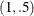and the linear constraints in a data set. Notice that the _TYPE_ variable contains keywords that identify how the procedure is to interpret each of the observations and that the parameters in the problems X1 and X2 are variables in the data set. The observation with _TYPE_=LOWERBD gives the lower bounds on the parameters. The observation with _TYPE_=GE gives the coefficients for the first constraint. Similarly, the subsequent observations contain specifications for the other constraints. Also notice that the special variable _RHS_ contains the right-hand-side values.

data betts1(type=est);
input _type_ $x1 x2 _rhs_; datalines; parms 1 .5 . lowerbd 0 0 . ge .57735 -1 . ge 1 1.732 . le 1 1.732 6 ;  Now you can solve this problem with the following code. Notice that you specify the objective function and the parameters. proc nlp inest=betts1 tech=trureg; min y; parms x1 x2; y = (((x1 - 3)**2 - 9) * x2**3) / (27 * sqrt(3)); run;  You can even include any constants used in the program statements in the INEST= data set. In the following code the variables A, B, C, and D contain some of the constants used in calculating the objective function Y. data betts2(type=est); input _type_$ x1    x2   _rhs_    a   b   c   d;
datalines;
parms    1        .5       .     3   9  27   3
lowerbd  0        0        .     .   .   .   .
ge       .57735   -1       0     .   .   .   .
ge       1        1.732    0     .   .   .   .
le       1        1.732    6     .   .   .   .
;


Notice that in the program statement for calculating Y, the constants are replaced by the A, B, C, and D variables.

proc nlp inest=betts2 tech=trureg;
min y;
parms x1 x2;
y = (((x1 - a)**2 - b) * x2**3) / (c * sqrt(d));
run;